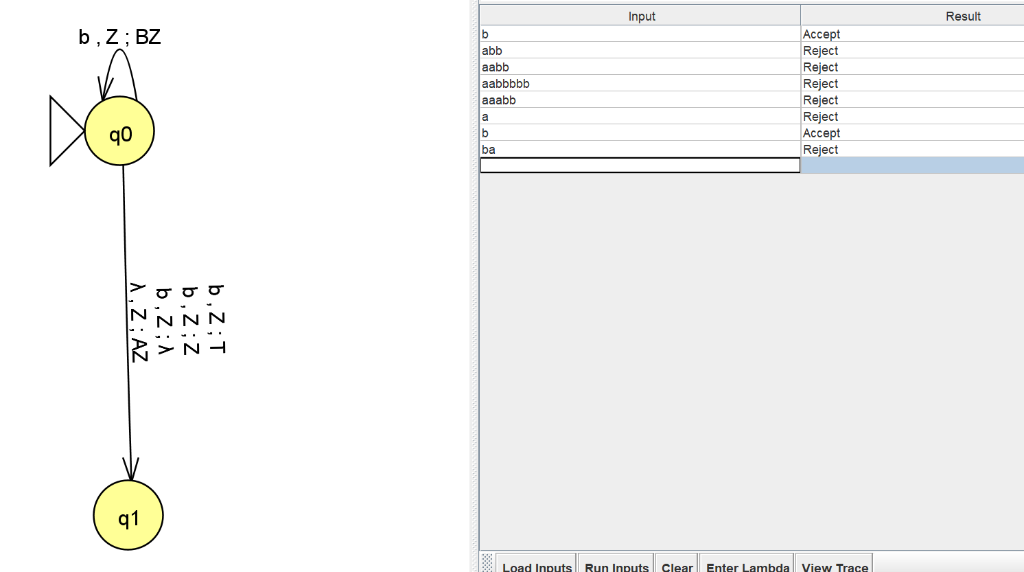Homework Help Question & Answers

# Q. Give one Trs example for a PDA (not found below) and add a comment in English next to it descr...

Q. Give one Trs example for a PDA (not found below) and add a comment in English next to it describing what it

means. If _____ then ______________.

Trs(q0, Ɛ, Z) = (q1, AZ) // push A

Trs(q0, b, Z) = (q0, BZ) // push B

Trs(q0, b, Z) = (q1, Ɛ) // pop Z

Trs(q0, b, Z) = (q1, Z) // replace Z with Z no change

Trs(q0, b, Z) = (q1, T) // pop Z and push T

#### Homework Answers

ReportAnswer #1The given PDA does not have any final stack so we assume that the string will be accepted when the stack is empty.

The only transition which leads to the empty stack is Trs(q0, b, Z) = (q1, Ɛ). So the PDA only accepts the string 'b'.

Add Homework Help Answer
##### Add Answer of: Q. Give one Trs example for a PDA (not found below) and add a comment in English next to it descr...
Your Answer: Your Name: What's your source?
Not the answer you're looking for? Ask your own homework help question. Our experts will answer your question WITHIN MINUTES for Free.
More Homework Help Questions Additional questions in this topic.

• #### which one of the following formulas accurately describes the meaning of this formula: (A5*B5)+10 A)add 10 to B5 and multiply the resulting value by A5 B)multiply A5 bY B5,then add 10 to the resulting value C)divide B5 into A5,then add 10 to the result D

Need Online Homework Help?

Get FREE EXPERT Answers
WITHIN MINUTES
Related Questions